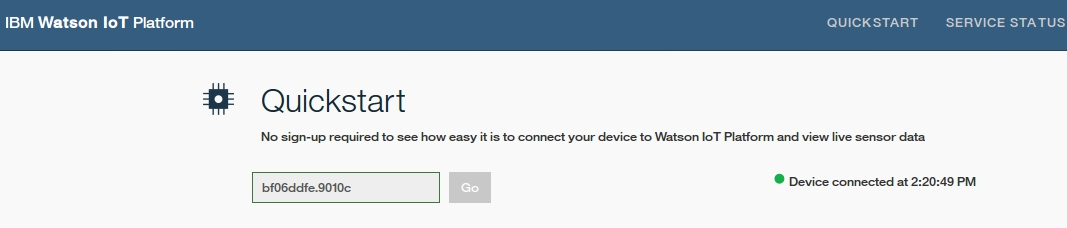## Sunday, August 20, 2017

### IBM Watson IoT (3): marble maze game with Raspberry Pi / Sense Hat and Node-RED

IBM WATSON IoT (3): MARBLE MAZE GAME WITH RASPBERRY PI / SENSE HAT AND NODE-RED

- Layout for this exercise1 - Introduction

- The goal of this exercise is to create a marble maze game using the Motion events properties (accelerometer, gyroscope, magenotmeter compass) and the 8x8 LED matrix provided by the Sense HAT module added on top of a Raspberry Pi device.

- The marble maze game consists of 3 types of colored and lighted dots: green (walls that cannot be traversed), red (one dot, what moves trying to find the 'goal') and blue (one dot, being the 'goal') .

- The rest of the dots (unlighted) in the matrix are the paths where the red dot moves along.

- The red dot moves trying to reach the position of the dot blue, at which point the goal of the game is achieved.

Once the goal of the game is achieved a new layout and goal position is automatically generated.

The Raspberry Pi device is connected to the Watson IoT platform where it sends events such as the final position (XY coordinates) of the blue dot each time the goal is achieved.

- This video shows how the game works:

2 - Creating a Node-RED flow

- The Node-RED flow consists of 4 nodes.

1) Sense Hat Output- This node is edited for using Motion events: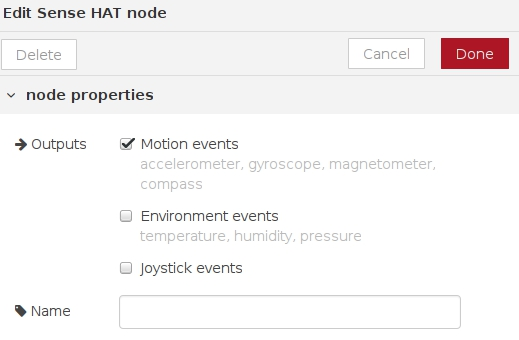2) Sense HAT Input- No special edition for this node:3) Function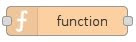- The node function generates the layout, the green walls, the red and blue dots, as well as collects the movement of the Raspberry Pi sensors:- JavaScript code of the function:

// New layout and goal position

function updateGoal() {
do {
goalX = Math.floor(Math.random()*7)+1;
goalY = Math.floor(Math.random()*7)+1;
} while(checkWall(goalX,goalY));

flow.set('goalX',goalX);
flow.set('goalY',goalY);
}

// Generating walls

function generateWalls() {
walls = [];
for (var i=0;i<10;i++) {
var wx = 0;
var wy = 0;
do {
wx = Math.floor(Math.random()*8);
wy = Math.floor(Math.random()*8);
} while(wx === x && wy === y);
walls.push({x:wx,y:wy});
}
flow.set('walls',walls);
}

// Checking collides with the walls

function checkWall(x,y) {
for (var i=0;i<walls.length;i++) {
if (walls[i].x === x && walls[i].y === y) {
return true;
}
}
return false;
}

// Retrieve positions

var x = context.get('x');
var y = context.get('y');

// Retrieve the current goal position from flow context

var goalX = flow.get('goalX');
var goalY = flow.get('goalY');

// Retrieve the current walls from flow context

var walls = flow.get('walls');

// Generate new walls if needed

if (!walls) {
generateWalls();
}

// Generate a new goal if needed

if (isNaN(goalX) || isNaN(goalY)) {
updateGoal();
}

var moved = false;
var ox = x;
var oy = y;

// Initializing the player position

if (isNaN(x) || isNaN(y)) {
ox = 3;
oy = 3;
x = 3;
y = 3;
moved = true;
} else {

// Retrieve the current roll and pitch from the message arriving from the SenseHAT

// Move the player based on tilt, ensuring it stays within the bounds of the screen

var sensitivity = 7;
if (roll > sensitivity && roll < 90) {
y += 1;
moved = true;
if (y > 6) { y = 7; }
} else if (roll < 360-sensitivity && roll > 270) {
y -= 1;
moved = true;
if (y < 1) { y = 0; }
}
if (pitch > sensitivity && pitch < 90) {
x -= 1;
moved = true;
if (x < 1) { x = 0; }
} else if (pitch < 360-sensitivity && pitch > 270) {
x += 1;
moved = true;
if (x > 6) { x = 7; }
}

// If the resultant position hits a wall, go back to where it started

if (checkWall(x,y)) {
x = ox;
y = oy;
}
}

// Store the new player position

context.set('x',x);
context.set('y',y);

if (moved) {
var eventMsg = null;

// If the player is on the goal, generate a new set of walls and goal position

if (x === goalX && y === goalY) {
generateWalls();
updateGoal();
// Blank the display
eventMsg = {
x: goalX,
y: goalY
}
};
} else {

// Blank the old position of the player

}

// Draw each of the walls

for (var i =0;i<walls.length;i++) {
}

// Draw the goal and player position

// Pass  the message to the Sense HAT Out node

return [msg,eventMsg];
}

// Nothing has moved so no need to update screen - return nothing

return null;

4) Watson IoT- The Watson IoT node is connected as a Device with the Quickstart service, what it is enough for the purpose of this exercise: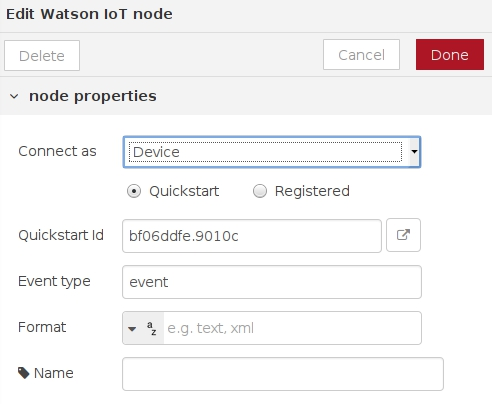- Deploying the flow: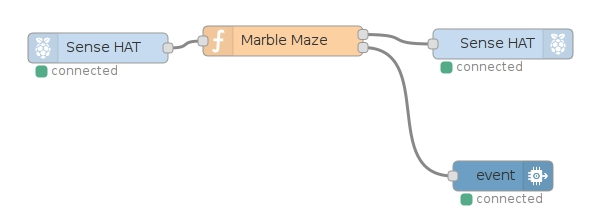- Clicking the Quickstart icon:- The Quickstart service offers information about the XY coordinates of the blue dot, located at the 8x8 LED matrix of the Sense HAT module, every time the goal is achieved: